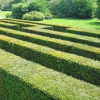#### You may also likeThis comes in two parts, with the first being less fiendish than the second. Itâ€™s great for practising both quadratics and laws of indices, and you can get a lot from making sure that you find all the solutions. For a real challenge (requiring a bit more knowledge), you could consider finding the complex solutions.### Discriminating

You're invited to decide whether statements about the number of solutions of a quadratic equation are always, sometimes or never true.This will encourage you to think about whether all quadratics can be factorised and to develop a better understanding of the effect that changing the coefficients has on the factorised form.

# The Circle of Apollonius... Coordinate Edition

##### Age 16 to 18Challenge LevelThis resource is from Underground Mathematics.

Two fixed points $A$ and $B$ lie in the plane, and the distance between them is $AB=2a$, where $a>0$.

A point $P$ moves in the plane so that the ratio of its distances from $A$ and $B$ is constant:
$$\frac{PA}{PB}=\lambda,$$
where $\lambda>0$.

1. Can you sketch the locus of the point $P$ for different values of $\lambda$?
2. Using Cartesian coordinates, work out (the equation of) the locus of $P$.

#### Suggestion

You may find it more straightforward to first work with specific values of $a$ and $\lambda$, say $a=2$ and $\lambda=3$.

#### Part 2

Now assuming that $\lambda\neq1$, find the radius and centre of the circle. What is the length of the tangent to this circle from the mid-point of $AB$? What shape is traced by the tangent as $\lambda$ varies?

#### Background

This circle is known as the circle of Apollonius, named after the Greek geometer Apollonius of Perga.

This is an Underground Mathematics resource.

Underground Mathematics is hosted by Cambridge Mathematics. The project was originally funded by a grant from the UK Department for Education to provide free web-based resources that support the teaching and learning of post-16 mathematics.

Visit the site at undergroundmathematics.org to find more resources, which also offer suggestions, solutions and teacher notes to help with their use in the classroom.